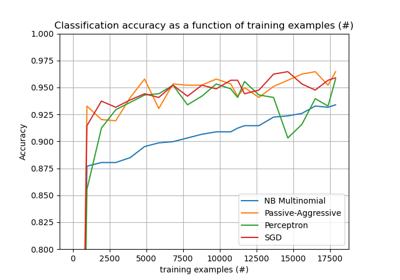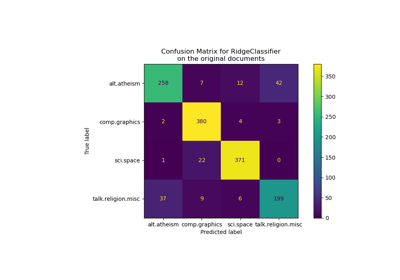# sklearn.linear_model.PassiveAggressiveClassifier¶

class sklearn.linear_model.PassiveAggressiveClassifier(C=1.0, fit_intercept=True, max_iter=1000, tol=0.001, early_stopping=False, validation_fraction=0.1, n_iter_no_change=5, shuffle=True, verbose=0, loss=’hinge’, n_jobs=None, random_state=None, warm_start=False, class_weight=None, average=False)[source]

Passive Aggressive Classifier

Read more in the User Guide.

Parameters: C : float Maximum step size (regularization). Defaults to 1.0. fit_intercept : bool, default=False Whether the intercept should be estimated or not. If False, the data is assumed to be already centered. max_iter : int, optional (default=1000) The maximum number of passes over the training data (aka epochs). It only impacts the behavior in the fit method, and not the partial_fit. New in version 0.19. tol : float or None, optional (default=1e-3) The stopping criterion. If it is not None, the iterations will stop when (loss > previous_loss - tol). New in version 0.19. early_stopping : bool, default=False Whether to use early stopping to terminate training when validation. score is not improving. If set to True, it will automatically set aside a stratified fraction of training data as validation and terminate training when validation score is not improving by at least tol for n_iter_no_change consecutive epochs. New in version 0.20. validation_fraction : float, default=0.1 The proportion of training data to set aside as validation set for early stopping. Must be between 0 and 1. Only used if early_stopping is True. New in version 0.20. n_iter_no_change : int, default=5 Number of iterations with no improvement to wait before early stopping. New in version 0.20. shuffle : bool, default=True Whether or not the training data should be shuffled after each epoch. verbose : integer, optional The verbosity level loss : string, optional The loss function to be used: hinge: equivalent to PA-I in the reference paper. squared_hinge: equivalent to PA-II in the reference paper. n_jobs : int or None, optional (default=None) The number of CPUs to use to do the OVA (One Versus All, for multi-class problems) computation. None means 1 unless in a joblib.parallel_backend context. -1 means using all processors. See Glossary for more details. random_state : int, RandomState instance or None, optional, default=None The seed of the pseudo random number generator to use when shuffling the data. If int, random_state is the seed used by the random number generator; If RandomState instance, random_state is the random number generator; If None, the random number generator is the RandomState instance used by np.random. warm_start : bool, optional When set to True, reuse the solution of the previous call to fit as initialization, otherwise, just erase the previous solution. See the Glossary. Repeatedly calling fit or partial_fit when warm_start is True can result in a different solution than when calling fit a single time because of the way the data is shuffled. class_weight : dict, {class_label: weight} or “balanced” or None, optional Preset for the class_weight fit parameter. Weights associated with classes. If not given, all classes are supposed to have weight one. The “balanced” mode uses the values of y to automatically adjust weights inversely proportional to class frequencies in the input data as n_samples / (n_classes * np.bincount(y)) New in version 0.17: parameter class_weight to automatically weight samples. average : bool or int, optional When set to True, computes the averaged SGD weights and stores the result in the coef_ attribute. If set to an int greater than 1, averaging will begin once the total number of samples seen reaches average. So average=10 will begin averaging after seeing 10 samples. New in version 0.19: parameter average to use weights averaging in SGD coef_ : array, shape = [1, n_features] if n_classes == 2 else [n_classes, n_features] Weights assigned to the features. intercept_ : array, shape =  if n_classes == 2 else [n_classes] Constants in decision function. n_iter_ : int The actual number of iterations to reach the stopping criterion. For multiclass fits, it is the maximum over every binary fit.

References

Online Passive-Aggressive Algorithms <http://jmlr.csail.mit.edu/papers/volume7/crammer06a/crammer06a.pdf> K. Crammer, O. Dekel, J. Keshat, S. Shalev-Shwartz, Y. Singer - JMLR (2006)

Examples

>>> from sklearn.linear_model import PassiveAggressiveClassifier
>>> from sklearn.datasets import make_classification

>>> X, y = make_classification(n_features=4, random_state=0)
>>> clf = PassiveAggressiveClassifier(max_iter=1000, random_state=0,
... tol=1e-3)
>>> clf.fit(X, y)
PassiveAggressiveClassifier(C=1.0, average=False, class_weight=None,
early_stopping=False, fit_intercept=True, loss='hinge',
max_iter=1000, n_iter_no_change=5, n_jobs=None,
random_state=0, shuffle=True, tol=0.001,
validation_fraction=0.1, verbose=0, warm_start=False)
>>> print(clf.coef_)
[[0.26642044 0.45070924 0.67251877 0.64185414]]
>>> print(clf.intercept_)
[1.84127814]
>>> print(clf.predict([[0, 0, 0, 0]]))



Methods

 decision_function(self, X) Predict confidence scores for samples. densify(self) Convert coefficient matrix to dense array format. fit(self, X, y[, coef_init, intercept_init]) Fit linear model with Passive Aggressive algorithm. get_params(self[, deep]) Get parameters for this estimator. partial_fit(self, X, y[, classes]) Fit linear model with Passive Aggressive algorithm. predict(self, X) Predict class labels for samples in X. score(self, X, y[, sample_weight]) Returns the mean accuracy on the given test data and labels. set_params(self, \*args, \*\*kwargs) sparsify(self) Convert coefficient matrix to sparse format.
__init__(self, C=1.0, fit_intercept=True, max_iter=1000, tol=0.001, early_stopping=False, validation_fraction=0.1, n_iter_no_change=5, shuffle=True, verbose=0, loss=’hinge’, n_jobs=None, random_state=None, warm_start=False, class_weight=None, average=False)[source]
decision_function(self, X)[source]

Predict confidence scores for samples.

The confidence score for a sample is the signed distance of that sample to the hyperplane.

Parameters: X : array_like or sparse matrix, shape (n_samples, n_features) Samples. array, shape=(n_samples,) if n_classes == 2 else (n_samples, n_classes) Confidence scores per (sample, class) combination. In the binary case, confidence score for self.classes_ where >0 means this class would be predicted.
densify(self)[source]

Convert coefficient matrix to dense array format.

Converts the coef_ member (back) to a numpy.ndarray. This is the default format of coef_ and is required for fitting, so calling this method is only required on models that have previously been sparsified; otherwise, it is a no-op.

Returns: self : estimator
fit(self, X, y, coef_init=None, intercept_init=None)[source]

Fit linear model with Passive Aggressive algorithm.

Parameters: X : {array-like, sparse matrix}, shape = [n_samples, n_features] Training data y : numpy array of shape [n_samples] Target values coef_init : array, shape = [n_classes,n_features] The initial coefficients to warm-start the optimization. intercept_init : array, shape = [n_classes] The initial intercept to warm-start the optimization. self : returns an instance of self.
get_params(self, deep=True)[source]

Get parameters for this estimator.

Parameters: deep : boolean, optional If True, will return the parameters for this estimator and contained subobjects that are estimators. params : mapping of string to any Parameter names mapped to their values.
partial_fit(self, X, y, classes=None)[source]

Fit linear model with Passive Aggressive algorithm.

Parameters: X : {array-like, sparse matrix}, shape = [n_samples, n_features] Subset of the training data y : numpy array of shape [n_samples] Subset of the target values classes : array, shape = [n_classes] Classes across all calls to partial_fit. Can be obtained by via np.unique(y_all), where y_all is the target vector of the entire dataset. This argument is required for the first call to partial_fit and can be omitted in the subsequent calls. Note that y doesn’t need to contain all labels in classes. self : returns an instance of self.
predict(self, X)[source]

Predict class labels for samples in X.

Parameters: X : array_like or sparse matrix, shape (n_samples, n_features) Samples. C : array, shape [n_samples] Predicted class label per sample.
score(self, X, y, sample_weight=None)[source]

Returns the mean accuracy on the given test data and labels.

In multi-label classification, this is the subset accuracy which is a harsh metric since you require for each sample that each label set be correctly predicted.

Parameters: X : array-like, shape = (n_samples, n_features) Test samples. y : array-like, shape = (n_samples) or (n_samples, n_outputs) True labels for X. sample_weight : array-like, shape = [n_samples], optional Sample weights. score : float Mean accuracy of self.predict(X) wrt. y.
sparsify(self)[source]

Convert coefficient matrix to sparse format.

Converts the coef_ member to a scipy.sparse matrix, which for L1-regularized models can be much more memory- and storage-efficient than the usual numpy.ndarray representation.

The intercept_ member is not converted.

Returns: self : estimator

Notes

For non-sparse models, i.e. when there are not many zeros in coef_, this may actually increase memory usage, so use this method with care. A rule of thumb is that the number of zero elements, which can be computed with (coef_ == 0).sum(), must be more than 50% for this to provide significant benefits.

After calling this method, further fitting with the partial_fit method (if any) will not work until you call densify.

## Examples using sklearn.linear_model.PassiveAggressiveClassifier¶Out-of-core classification of text documentsComparing various online solversClassification of text documents using sparse features